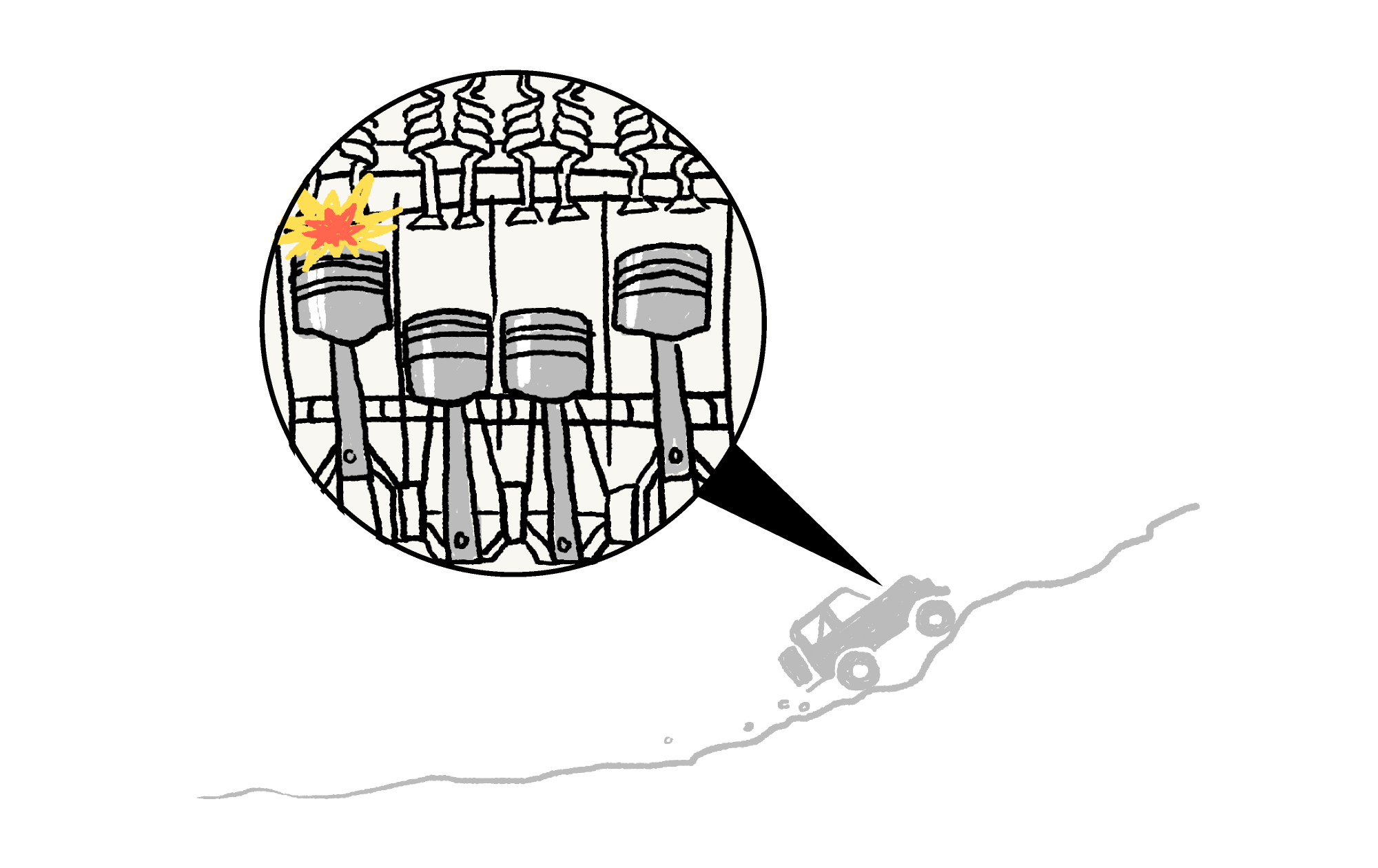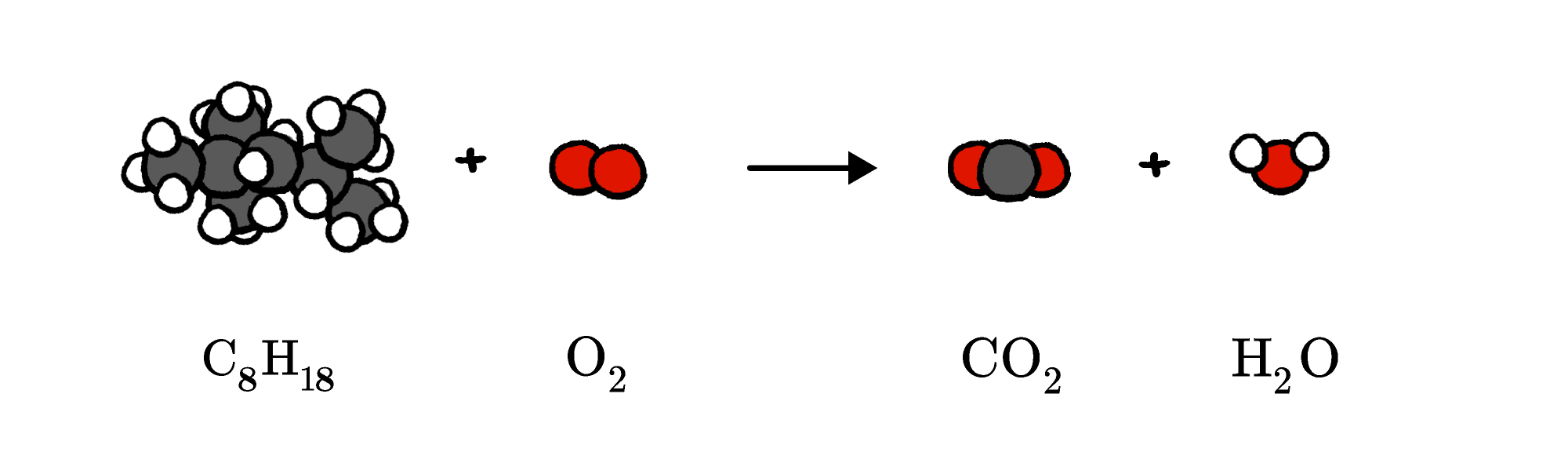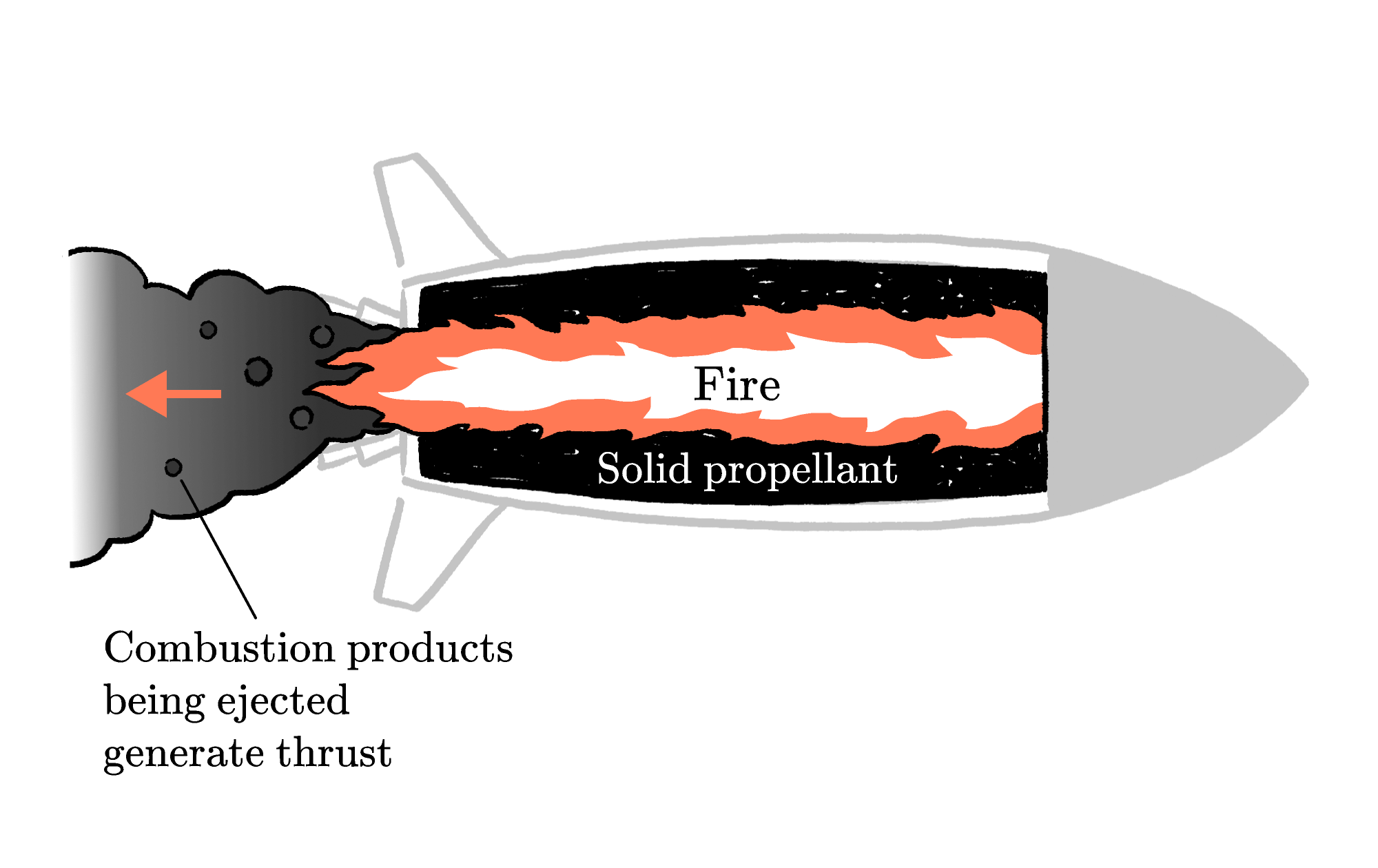Back

## Rocket Fuel Reaction

The vast majority of cars need to burn fuel in order to operate, but how does fuel store energy and how does burning release it?Chemical energy is stored in molecular bonds, and burning fuel breaks those bonds, freeing up some of the energy to power your car.

Consider octane $(\ce{C8H18}),$ an important component of the gasoline used in cars. When octane reacts with oxygen, the energy in bonds is released and the atoms reform into new molecules with lower energy bonds — $\ce{CO2}$ and $\ce{H2O}$ — while the excess bond energy is released as heat."But wait," you might protest, "between octane and $\ce{O2}$ there are $2$ oxygen atoms, but between carbon dioxide and water there are $3.$" If this seems improper to you, you're right, and that's why we can't be satisfied with merely knowing the identity of reactants.

Reactions can't create, destroy, or transform the identity of atoms — we're doing chemistry, not alchemy, after all.

This equation has the right chemicals present, but we need to do some arithmetic to balance it. We need to figure out how many of each chemical species we need so that the total number of each atom is the same on each side of the equation. Since octane is the most constrained molecule $($it always has $8$ carbon atoms for every $18$ hydrogen atoms$),$ we'll try to use one of it and find the number of each of the other molecules we need to balance it: $\ce{C8H18 + xO2 -> yH2O + zCO2}.$ To find the other coefficients, we can break the equation down element by element. For example, since octane $(8$ carbon atoms per molecule$)$ and carbon dioxide $(1$ carbon atom per molecule$)$ are the only carbon-containing molecules, if the carbon atoms are going to balance them, it has to be the case that $z=8.$ Applying similar logic to hydrogen and oxygen, we get \begin{aligned} 18 &= 2y \\ 2x &= y + 2z. \end{aligned} The hydrogen equation shows that $y = 9,$ which we can plug into the oxygen equation $($along with $z = 8)$ to get $x = 12.5,$ which leads to $\ce{C8H18 + 12.5O2 -> 9H2O + 8CO2}.$ Not many people like decimals, and even fewer people like half oxygen molecules, so we can multiply the whole thing by $2$ to get our final balanced equation for the combustion of octane: $\ce{2C8H18 + 25O2 -> 18H2O + 16CO2}.$

# Today's Challenge

The fuel used to propel spaceships can't depend on atmospheric oxygen as a reactant, since they might not be operating in the atmosphere! Another option is to use solid propellants, where both the fuel and oxidizer are present in the propellant.One type of solid propellant burns aluminum and ammonium perchlorate $(\ce{NH4ClO4}).$ This is a complex reaction with many potential products, but one possible (unbalanced) reaction is this: \begin{aligned} \ce{Al + NH4ClO4 -> \ & Al2O3 + AlCl3 \\ &+ N2 + H2O}. \end{aligned} The mass of a molecule of $\ce{NH4ClO4}$ is about $4$ times the mass of an atom of $\ce{Al}$. If you're making a rocket engine that uses aluminum and ammonium perchlorate as its solid propellant, what percent of the propellant should be aluminum by mass?×